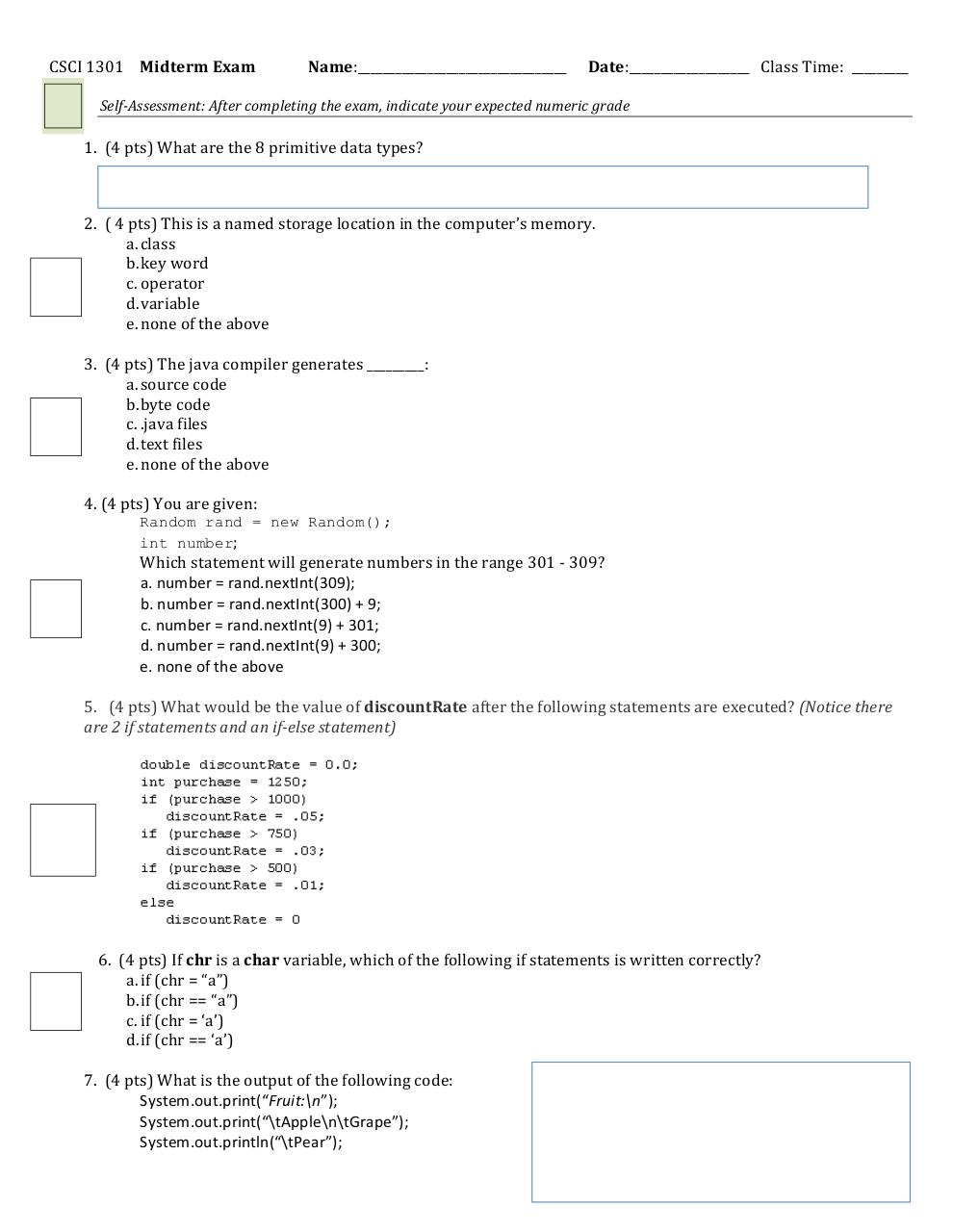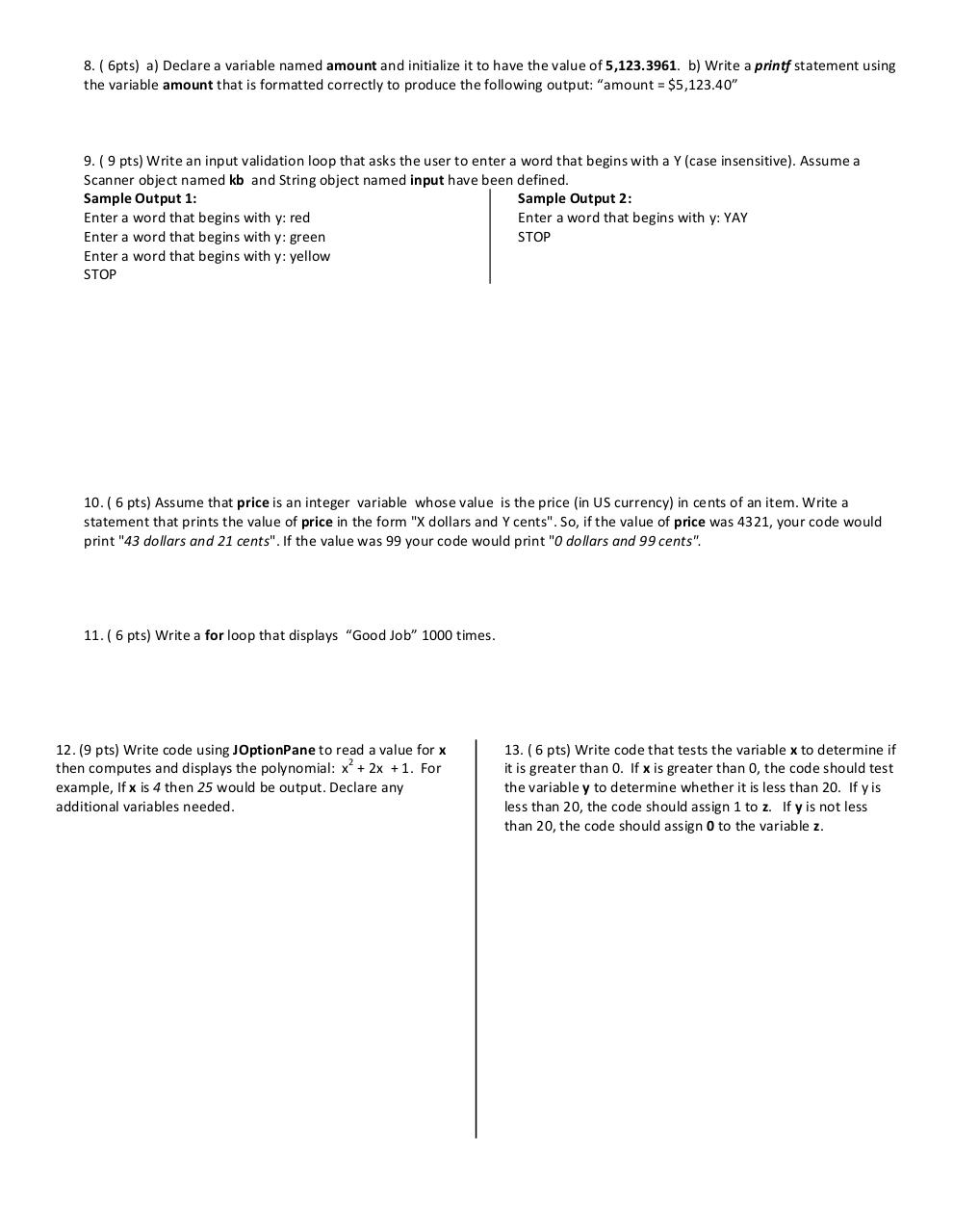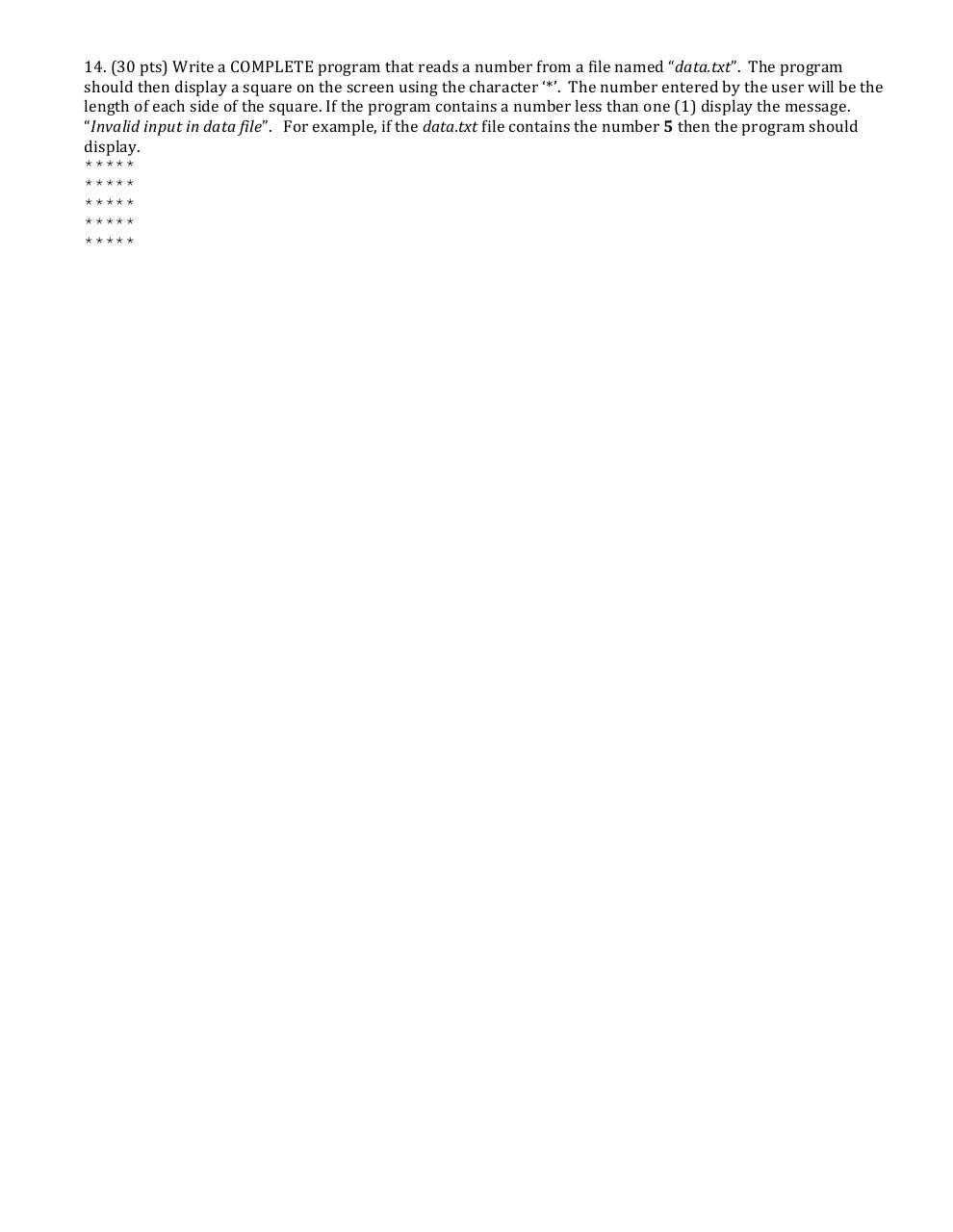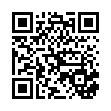# 1301Midterm F2015 .pdf

### File information

Original filename: 1301Midterm_F2015.pdf
Title: Microsoft Word - 1301Midterm_F2015.docx

This PDF 1.3 document has been generated by Word / Mac OS X 10.9.3 Quartz PDFContext, and has been sent on pdf-archive.com on 04/10/2015 at 21:41, from IP address 168.16.x.x. The current document download page has been viewed 782 times.
File size: 144 KB (3 pages).
Privacy: public file

1301Midterm_F2015.pdf (PDF, 144 KB)

### Document preview

CSCI  1301   Midterm  Exam

Name:_________________________________

Date:___________________      Class  Time:    _________

1.    (4  pts)  What  are  the  8  primitive  data  types?

2.    (  4  pts)  This  is  a  named  storage  location  in  the  computer’s  memory.
a. class
b. key  word
c. operator
d. variable
e. none  of  the  above

3.    (4  pts)  The  java  compiler  generates  _________:
a. source  code
b. byte  code
c. .java  files
d. text  files
e. none  of  the  above

4.  (4  pts)  You  are  given:
Random rand = new Random();
int number;

Which  statement  will  generate  numbers  in  the  range  301  -­‐  309?
a.  number  =  rand.nextInt(309);

b.  number  =  rand.nextInt(300)  +  9;

c.  number  =  rand.nextInt(9)  +  301;

d.  number  =  rand.nextInt(9)  +  300;

e.  none  of  the  above

5.      (4  pts)  What  would  be  the  value  of  discountRate  after  the  following  statements  are  executed?  (Notice  there
are  2  if  statements  and  an  if-­‐else  statement)

6.    (4  pts)  If  chr  is  a  char  variable,  which  of  the  following  if  statements  is  written  correctly?
a. if  (chr  =  “a”)
b. if  (chr  ==  “a”)
c. if  (chr  =  ‘a’)
d. if  (chr  ==  ‘a’)

7.    (4  pts)  What  is  the  output  of  the  following  code:
System.out.print(“Fruit:\n”);
System.out.print(“\tApple\n\tGrape”);
System.out.println(“\tPear”);

8.  (  6pts)    a)  Declare  a  variable  named  amount  and  initialize  it  to  have  the  value  of  5,123.3961.    b)  Write  a  printf  statement  using
the  variable  amount  that  is  formatted  correctly  to  produce  the  following  output:  “amount  =  \$5,123.40”

9.  (  9  pts)  Write  an  input  validation  loop  that  asks  the  user  to  enter  a  word  that  begins  with  a  Y  (case  insensitive).  Assume  a
Scanner  object  named  kb    and  String  object  named  input  have  been  defined.
Sample  Output  1:
Sample  Output  2:
Enter  a  word  that  begins  with  y:  red
Enter  a  word  that  begins  with  y:  YAY
Enter  a  word  that  begins  with  y:  green
STOP
Enter  a  word  that  begins  with  y:  yellow
STOP

10.  (  6  pts)  Assume  that  price  is  an  integer    variable    whose  value    is  the  price  (in  US  currency)  in  cents  of  an  item.  Write  a
statement  that  prints  the  value  of  price  in  the  form  "X  dollars  and  Y  cents".  So,  if  the  value  of  price  was  4321,  your  code  would
print  "43  dollars  and  21  cents".  If  the  value  was  99  your  code  would  print  "0  dollars  and  99  cents".

11.  (  6  pts)  Write  a  for  loop  that  displays    “Good  Job”  1000  times.

12.  (9  pts)  Write  code  using  JOptionPane  to  read  a  value  for  x
13.  (  6  pts)  Write  code  that  tests  the  variable  x  to  determine  if
2
then  computes  and  displays  the  polynomial:    x  +  2x    +  1.    For
it  is  greater  than  0.    If  x  is  greater  than  0,  the  code  should  test
example,  If  x  is  4  then  25  would  be  output.  Declare  any
the  variable  y  to  determine  whether  it  is  less  than  20.    If  y  is
less  than  20,  the  code  should  assign  1  to  z.      If  y  is  not  less

than  20,  the  code  should  assign  0  to  the  variable  z.

14.  (30  pts)  Write  a  COMPLETE  program  that  reads  a  number  from  a  file  named  “data.txt”.    The  program
should  then  display  a  square  on  the  screen  using  the  character  ‘*’.    The  number  entered  by  the  user  will  be  the
length  of  each  side  of  the  square.  If  the  program  contains  a  number  less  than  one  (1)  display  the  message.
“Invalid  input  in  data  file”.      For  example,  if  the  data.txt  file  contains  the  number  5  then  the  program  should
display.
*****
*****
*****
*****
*****#### HTML Code

Copy the following HTML code to share your document on a Website or Blog

#### QR Code### Related keywords